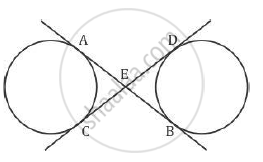# common tangents AB and CD to the two circles with centres 01and 02 intersect at E. Prove that AB = CD. - Mathematics

In Figure 1, common tangents AB and CD to the two circles with centres 01and 0intersect at E. Prove that AB = CD.#### SolutionGiven: AB and CD are common tangents to both the circles.
To prove: AB = CD
Proof:
We know that two tangents drawn to a circle for the same exterior point are
equal.
Thus we get
AE = EC (i)
Similarly
ED = EB (ii)
AB = AE + EB (iii)
and
CD = CE + ED (iv)
AB = EC + EB from (i) and (iii)
CD = EC + EB from (ii) and (iv)
Therefore AB = CD
Hence proved.

Concept: Concept of Circle - Centre, Radius, Diameter, Arc, Sector, Chord, Segment, Semicircle, Circumference, Interior and Exterior, Concentric Circles
Is there an error in this question or solution?
2013-2014 (March) All India Set 2

Share